# Coaching Materials in Satellite Communications Part 1 for ECE Board Exam

(Last Updated On: December 8, 2017)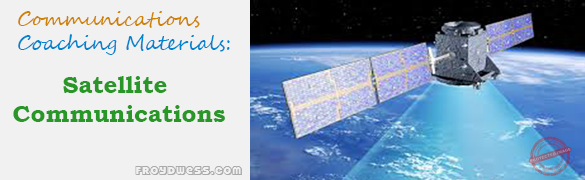This is the Coaching materials in Satellite Communications Part 1 as one topic in ECE Board Exam taken from various sources including but not limited to past Board Examination Questions in Electronic System and Technologies (EST), Communications Books, Journals and other Communications References. This particular Coaching Notes in Communications Engineering has random Questions and Answers in random topics. Make sure to familiarize this review notes to increase the chance of passing the ECE Board Exam.

### Satellite Communications Coaching Materials Part 1

1. What are the two types of communications satellite

Passive and active

2. A typical satellite communications operational link consists of a satellite and what other two components?

Earth terminals

3. What areas of the earth are not normally covered by satellites?

Extreme polar regions

4. Satellite orientation in space is important for what two reasons?

To allow maximum solar exposure to the sun and satellite antenna exposure to earth terminals

5. What types of antennas are generally used at earth terminals?

Large, high-gain parabolic antennas

6. Why do earth terminals require highly sensitive receivers?

To overcome satellite transmitter low power and permit extraction of the desired information from the received signal

7. Why is satellite acquisition and tracking important?

To ensure earth terminal antennas are always pointed towards the satellite

8. What are the two limitations to an active satellite communications system?

9. If the line-of-sight distance for an optical beam is 12 km, what would is be, approximately, for a microwave beam?

16 km

10. The power in the downlink signal from a typical communications satellite is in the range of _____ per transponder.

10 to 250 watts

11. The power level for an earth station to transmit to a satellite is in the order of:

10^3 watts

12. Ku-band antennas can be _____ than C-band antennas.

Smaller

13. A geosynchronous orbit is about _____ km above the earth.

35,780

14. A Global Positioning System (GPS) or NAVSTAR is

MEO

15. GPS or NAVSTAR height is

9500 miles

16. An antenna’s angular direction between east and west?

Azimuth

17. An antenna’s vertical angle with respect to the earth’s surface.

Elevation

18. An antenna’s angle by which it is offset from the earth’s axis

Declination

19. In geostationary satellites, “station-keeping” means:

20. A reduction of TWT power for linearity is called:

Backoff

21. The frequency bands used by Ku-band satellites are:

12 GHz and 14 GHz

22. Satellite operating at 27 – 30 GHz

Ka-band

23. The law that states that a satellite will orbit a primary body following an elliptical path.

1st Law of Kepler

24. Kepler’s 2nd law is known as

Law of areas

25. Kepler’s 3rd law is also known as

Harmonic law

26. Low Earth Orbit (LEO) satellite orbits around the earth at a height of

370 miles

27. Medium Earth Orbit (MEO) satellite orbits around the earth at a height approximately _____.

6000 miles to 12,000 miles

28. Geosynchronous satellites are high-altitude earth-orbit satellites with heights about _____.

22,300 miles or 36,000 km

29. Iridium is a LEOSAT that has a height of approximately

480 miles

30. MEO frequency band is

1.2 -1.66 GHz

31. All satellites rotate around the earth in an orbit that forms a plane that passes through the center of gravity of earth called

Geocenter

32. The point on the surface of the earth directly below the satellite

Subsatellite point (SSP)

33. The minimum acceptance angle of elevation

5 degrees

34. The spatial separation of a satellite is between _____.

3 degrees to 6 degrees

35. Antenna used with a rotating satellite whose main beam is continuously adjusted so that it will illuminate a given area on the earth’s surface

Despun

36. Satellite’s radiation pattern that typically target up to 20% of the Earth’s surface

Hemispherical beams

37. The radiation pattern that has a satellite’s antenna beamwidth of 17 degrees and are capable of covering approximately 42% of the earth’s surface

Earth or global beams

38. Geostats has an earth coverage of approximately

42.5%

39. LNA stands for:

Low-noise amplifier

40. LNA in satellite transponder system commonly used

Tunnel

41. Find the velocity of a satellite in a circular orbit (a) 500 km above the earth’s surface (b) 36,000 km above the earth’s surface (approximately the height of geosynchronous satellite).

(a) 7.6 km/s (b) 3.07 km/s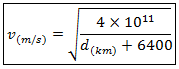42. Calculate the angle of declination for an antenna using a polar mount at latitude of 45 degrees.

6.81 degrees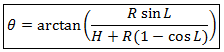43. Calculate the length of the path to a geosynchronous satellite from an earth station where the angle of elevation is 30 degrees.

39 x 10^3 km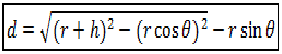44. A satellite transmitter operates at a 4 GHz with a transmitter power of 7W and an antenna gain of 40 dBi. The receiver has antenna gain of 30 dBi and the path length is 40,000 km. Calculate the signal strength at the receiver.

-88 dBm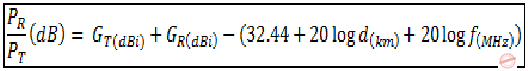45. A receiving antenna with a gain of 40 dBi looks at at a sky with a noise temperature of 15K. The loss between the antenna and the LNA input, due to the feedhorn, is 0.4 dB, and the LNA has a noise temperature of 40K. Calculate G/T (Antenna gain-to-noise-temperature).

20.6 dB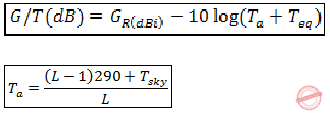46. A receiver has a noise figure of 1.5 dB. Find its equivalent noise temperature.

119K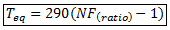47. The receiving installation whose G/T was found to be 20.6 dB is used as a ground terminal to receive a signal from a satellite at a distance of 38,000 km. the satellite has transmitter power of 50 watts and an antenna gain of 30 dBi. Assume losses between the satellite transmitter and its antenna is negligible. The frequency is 12 GHz. Calculate the carrier-to-noise ratio at the receiver, for a bandwidth of 1 MHz.

30.6 dB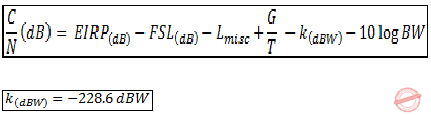48. If a satellite has a total transmitter power (Pt) of 1000 W, determine the energy per bit (Eb) for a transmission rate of 50 Mbps.

-47 dBW/bps or -47 dbJ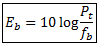49. For an equivalent noise bandwidth of 10 MHz and a total noise power of 0.00276 pW, determine the noise density and equivalent noise temperature.

276 x 10^-23 W/Hz or -205.6 dBW/Hz

50. A typical TVRO installation for use with C-band satellite (downlink at approximately 4 GHz) has a diameter of about 3 m and an efficiency of about 55%. Calculate its gain and beamwidth.

G = 39 dB, beamwidth = 1.75 degrees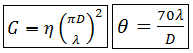51. For an earth station transmitter with an antenna output power of 40dB (10000 W), a back-off loss of 3dB, a total branching and feeder loss of 3dB, and a transmitter antenna gain of 40dB, determine the EIRP

74 dBW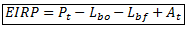Rate this: